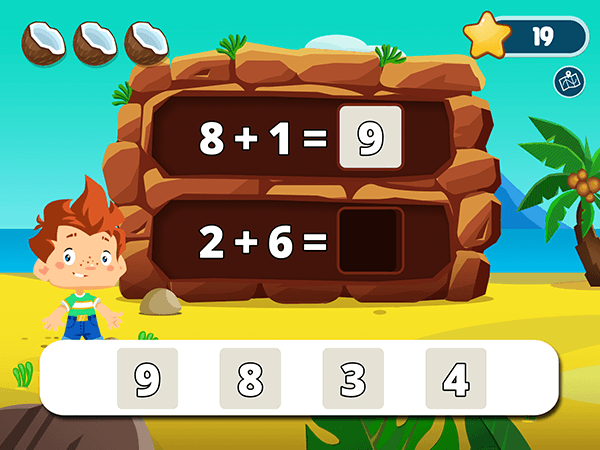• Make a number within 10
• Addition within 18 with and without regrouping
• Addition within 18 - vertical
• Make a number within 18 with and without regrouping
• and many more...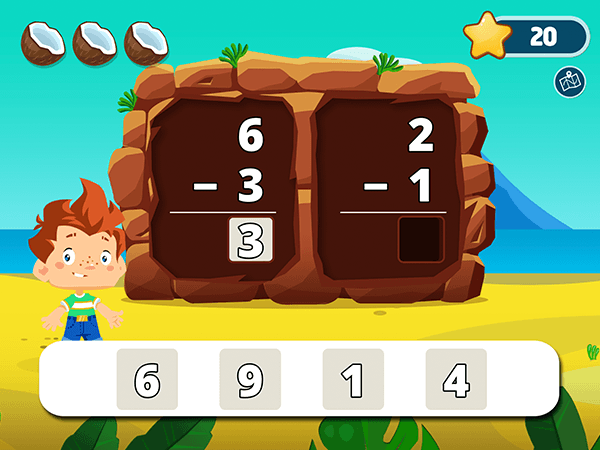Subtraction
• Subtraction within 10
• Subtraction within 10 - vertical
• Make a number within 10
• Subtraction within 18 with and without regrouping
• Subtraction within 18 - vertical
• Make a number within 18 with and without regrouping
• and many more...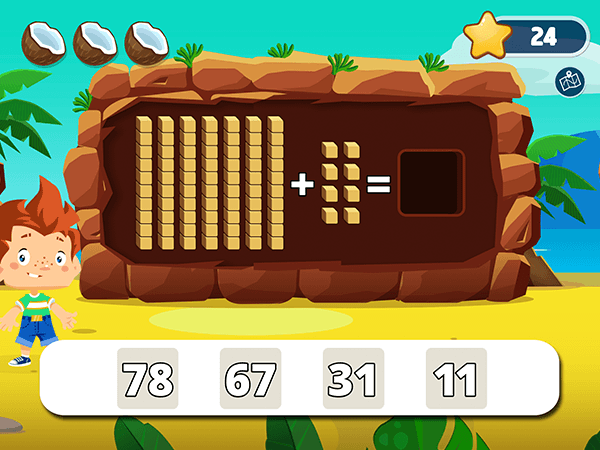Place Value
• Addition within 10 with cubes
• Number line to 10 & 20
• Base 10 with cubes
• Multiples of 10
• Tens and ones with cubes
• Add ones to tens with cubes
• Place the missing number in the table
• and many more...Geometry
• Identify the 2D shape
• Count & Compare sides and vertexes
• 3D shapes
• Identify the equal shares
• Halves
• Thirds
• Fourths
• and many more...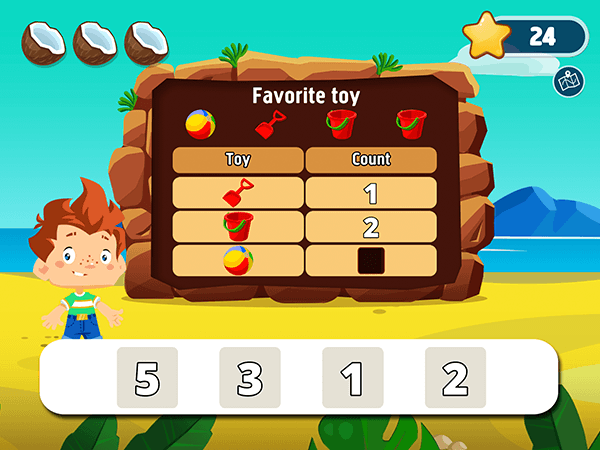Measurements and Data
• Complete Table Data
• The Analog Clock
• Telling Time to the Hour
• Match digital clocks and times
• Objects by Length
• and many more...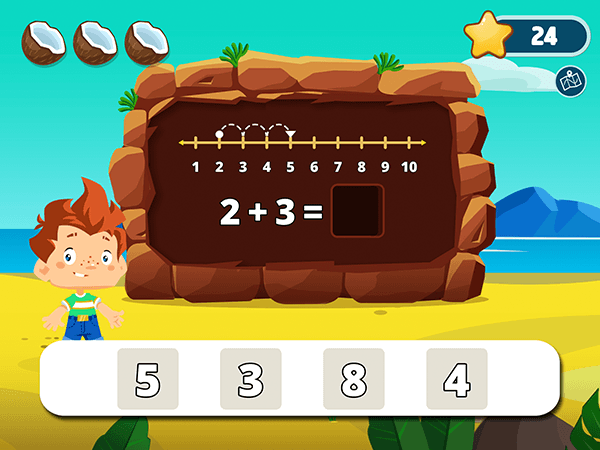• Count forward using a number line
• Making 10
• Doubles
• Doubles plus 1
• Add in any order within 10 & 20
• and many more...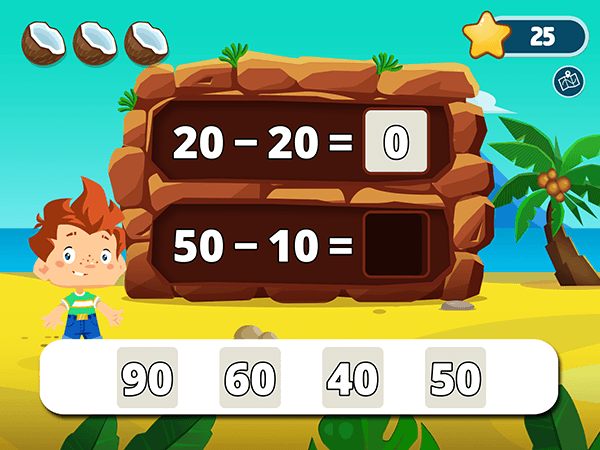Subtraction Strategies
• Count backward using a number line
• Subtract zero
• Subtract the same number
• Doubles
• Subtract multiples of 10
• and many more...Counting and Comparing
• Odd or Even within 20 & 100
• Compare Cubes
• Compare numbers up to 10 & 100
• Compare numbers in multiples of 10
• Count by 1s, 2s, 5s, 10s
• and many more...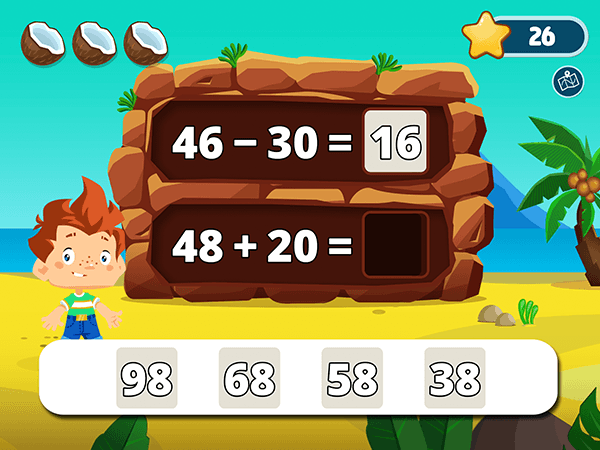Mixed Operations
• Addition and subtraction within 10 & 20
• Add or subtract within 10 & 20
• Make a number within 20
• Add and subtract a multiple of 10